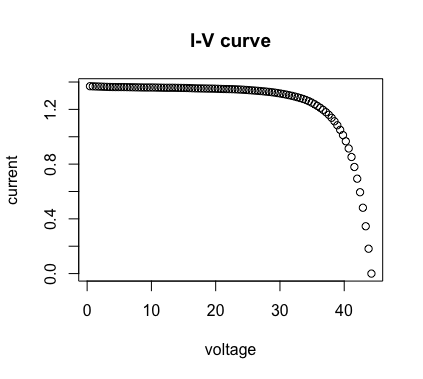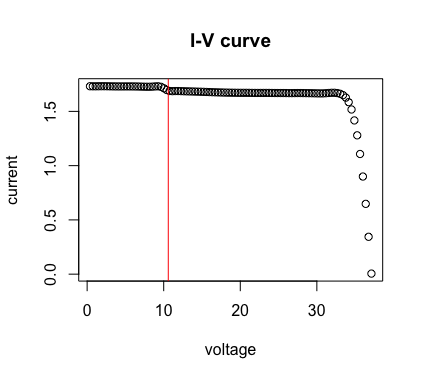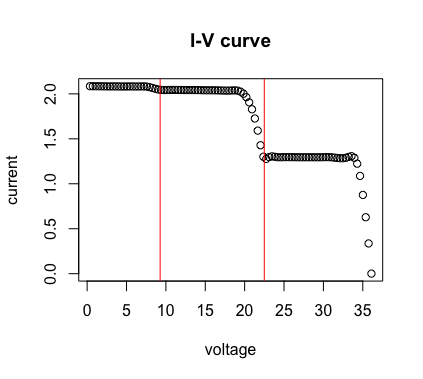ddiv v.0.1.1

2021-04-14

This information is based off the following paper. X. Ma, W. Huang, E. Schnabel, M. Köhl, J. Brynjarsdóttir, J. L. Braid, and R. H. French. “Data-Driven I–V Feature Extraction for Photovoltaic Modules.” IEEE Journal of Photovoltaics 9, no. 5 (September 2019): 1405–12. https://doi.org/10.1109/JPHOTOV.2019.2928477. —————————

What is ddiv v0.1.1 and what does it do?

The R package “ddiv v0.1.1” use data driven method to extract $$I-V$$ features for given $$I-V$$ curves. This method aims to apply the basic definitions of the eight parameters (which is introduced in the vignette file “IVcurve”) automatically calculate their values for large numbers of $$I-V$$ curves. For a given $$I-V$$ curve, the this package first identify steps in $$I-V$$ curves (definition of steps is introduced in the vigette file “IVcurve”), then extract $$I-V$$ features for each steps of $$I-V$$ curves.

Segmented regression is used to find change points in $$I-V$$ curves. Here we apply “Segmented Regression” package and pull out the slopes for each range of data partitioned by this package. As segmented regression only help to identify change points in a curve, but not all change points indicates steps in $$I-V$$ curves. Thus we add several more criterion for the slopes we pulled out to identify the change points indicating steps. After calculate the steps in $$I-V$$ curves, we partition the whole data points into subsets for different steps of $$I-V$$ curves. We treat each of these different steps of $$I-V$$ curve as a single $$I-V$$ curve to extract features introduced below.

With the definitions of the eight $$I-V$$ features, while most of the features are straightforward, calculations for $$I_{sc}$$ and $$R_{sh}$$ are more complex. The first step in determining $$I_{sc}$$ and $$R_{sh}$$ is to run a linear regression with current being dependent variable and voltage being independent variable for a moving window of five consecutive points on $$I-V$$ curve, and store the slopes for every regression. The the change of slope is calculated. The change in slope indicates a change in shape along the $$I-V$$ curve. The change in slope remains relatively stable for the linear part near $$I_{sc}$$ where voltages are small. Thus we set a critical value (which is indicated by variable “crt” in IVExtractResult function) for change in slope to find this linear region and pull out the corresponding current and voltage points. With the selected data points, a linear regression is used to find the slope and intercept for the linear region of the $$I-V$$ curve near $$I_{sc}$$. In accordance with the definitions of $$I_{sc}$$ and $$R_{sh}$$, $$I_{sc}$$ is estimated with the intercept of the fitted line, and $$R_{sh}$$ is estimated by the inverse of its slope. As each $$I-V$$ curve may behave differently with various magnitudes of slope values, and different changing points to curvature. With this method, we can automatically find the appropriate current and voltage points for the front linear part of $$I-V$$ curves.

$$V_{oc}$$ and $$R_{s}$$ are calculated from the linear part in the $$I-V$$ curve above $$P_{mp}$$. When observing the dataset, there are generally fewer data points in the linear region near $$V_{oc}$$ than the one near $$I_{sc}$$. The points that have very large change in current value are corresponding to the linear part near $$V_{oc}$$. Thus we set a critical value (which is indicated by the variable “crtvalb” in IVExtractResult Function) and select the data points that have current change larger than the critical value. According to the definition of $$V_{oc}$$ and $$R_{s}$$, a regression is run with voltage being the dependent variable and current being the independent variable. Then $$V_{oc}$$ is estimated by the linear intercept, and $$R_{s}$$ is estimated by the slope. $$P_{mp}$$, $$FF$$, $$I_{mp}$$ and $$V_{mp}$$ are straightforward to calculate after $$V_{oc}$$ and $$I_{sc}$$ are estimated using the definitions introduced in vignette file “IVcurve”.

How does ddiv v0.1.0 work?

The R package “ddiv v0.1.0” has three main functions. The general process to extract IV features for a given $$I-V$$ curve is to first identify steps in $$I-V$$ curves. Then for each steps of $$I-V$$ curve, the eight $$I-V$$ features are extracted.

“IVsteps()” is the function to identify steps in $$I-V$$ curves. It uses the “Segemented Regression” package and our added criterion to find change points and test if the change points are indication for steps. This function gives the number of steps in an $$I-V$$ curve (step), and the change points indicating steps (xsep). If this function returns “step=1”, it means the $$I-V$$ curve is of one step, which is the standard $$I-V$$ curve, so there will be no change points indicating steps, thus “xsep” would be “NA”. And if the function returns “step=2”, we will have “xsep” giving us 1 change points, and so on.

“IVfeature()” is the function to extract $$I-V$$ features for a standard $$I-V$$ curve ($$I-V$$ curve with one step). This function returns the values for the extracted eight $$I-V$$ features. If we are extracting $$I-V$$ curves that are known to have perfect standard shape, which are one step $$I-V$$ curves, we could directly use this function without identifying the number of steps and location of change points in $$I-V$$ curves. If $$I-V$$ curves are having difference shapes, with varying step numbers, it would be better to use the “IVExtractResult()” function.

“IVExtractResult()” is the main function in “ddiv v0.1.0” package. This funtion first use “IVsteps()” function to identify number of steps and location of change points. Then, if the $$I-V$$ curve has only one step, then “IVfeature()” function is applied to the whole $$I-V$$ curve; if the $$I-V$$ curve has more than one steps, it partition the whole $$I-V$$ curve into small standard $$I-V$$ curves. For each small standard $$I-V$$ curves, “IVfeature()” function is applied to extract eight $$I-V$$ features. In total, $$n$$ sets of $$I-V$$ features will be extracted, and $$n$$ is the number of steps in the $$I-V$$ curve. Thus this function will return the number of steps $$n$$, location of change points, and $$n$$ sets of the eight $$I-V$$ features.

install.packages("ddiv_0.1.0.tar.gz", repos = NULL, type = "source")
library(ddiv)

We put three example $$I-V$$ curves in this package. They are $$I-V$$ curves with one step, two steps, and three steps.

Example code for ddiv v0.1.0

## Load the IV dataset with one step
data(IV_step1)
IV1 <- data.frame(IV_step1)

## Run IVExtractResult
IVExtractResult(IV1,plot.option=TRUE)

## As this IV curve is known to have one step, IVfeature() can be used
IVfeature(IV1$I,IV1$V,crt=0.2,num=75,crtvalb=0.3)

## Load the IV dataset with two steps
data(IV_step2)
IV2 <- data.frame(IV_step2)

## Run IVExtractResult
IVExtractResult(IV2,plot.option=TRUE)

## Load the IV dataset with three steps
data(IV_step3)
IV3 <- data.frame(IV_step3)

## Run IVExtractResult
IVExtractResult(IV3,plot.option=TRUE)

Example output for ddiv v0.1.0The result for finding steps for example data “IV_step1”, no changepoint identifying step is foundThe result for finding steps for example data “IV_step2”, the red line is the changepoint indicating step in IV curveThe result for finding steps for example data “IV_step3”, the red lines are the changepoints indicating steps in IV curves

Sources

1. Ma, Xuan. “Data-Driven Current-Voltage Feature Extraction And Time Series Analysis for Mechanistic Photovoltaic Module Degradation”. Electronic Thesis or Dissertation. Case Western Reserve University, 2018. OhioLINK Electronic Theses and Dissertations Center. May 2018.

2. Timothy J Peshek, Justin S Fada, Yang Hu, Yifan Xu, Mohamed A Elsaeiti, Erdmut Schnabel, Michael Köhl, and Roger H French. Insights into metastability of photovoltaic materials at the mesoscale through massive i–v analytics. Journal of Vacuum Science & Technology B, Nanotechnology andMicroelectronics: Materials, Processing, Measurement, and Phenomena, 34(5):050801, 2016.